# 雜湊表（Hash Table）

「雜湊表（hash table）」，又可稱為「哈希表」，是透過鍵（key）值找到資料在記憶體位置的儲存方式。將數據透過雜湊函式（hash function）映射（map）到其在表中的對應位置後，可同步降低操作時的「空間複雜度」與「時間複雜度」。

## 雜湊函式（hash function）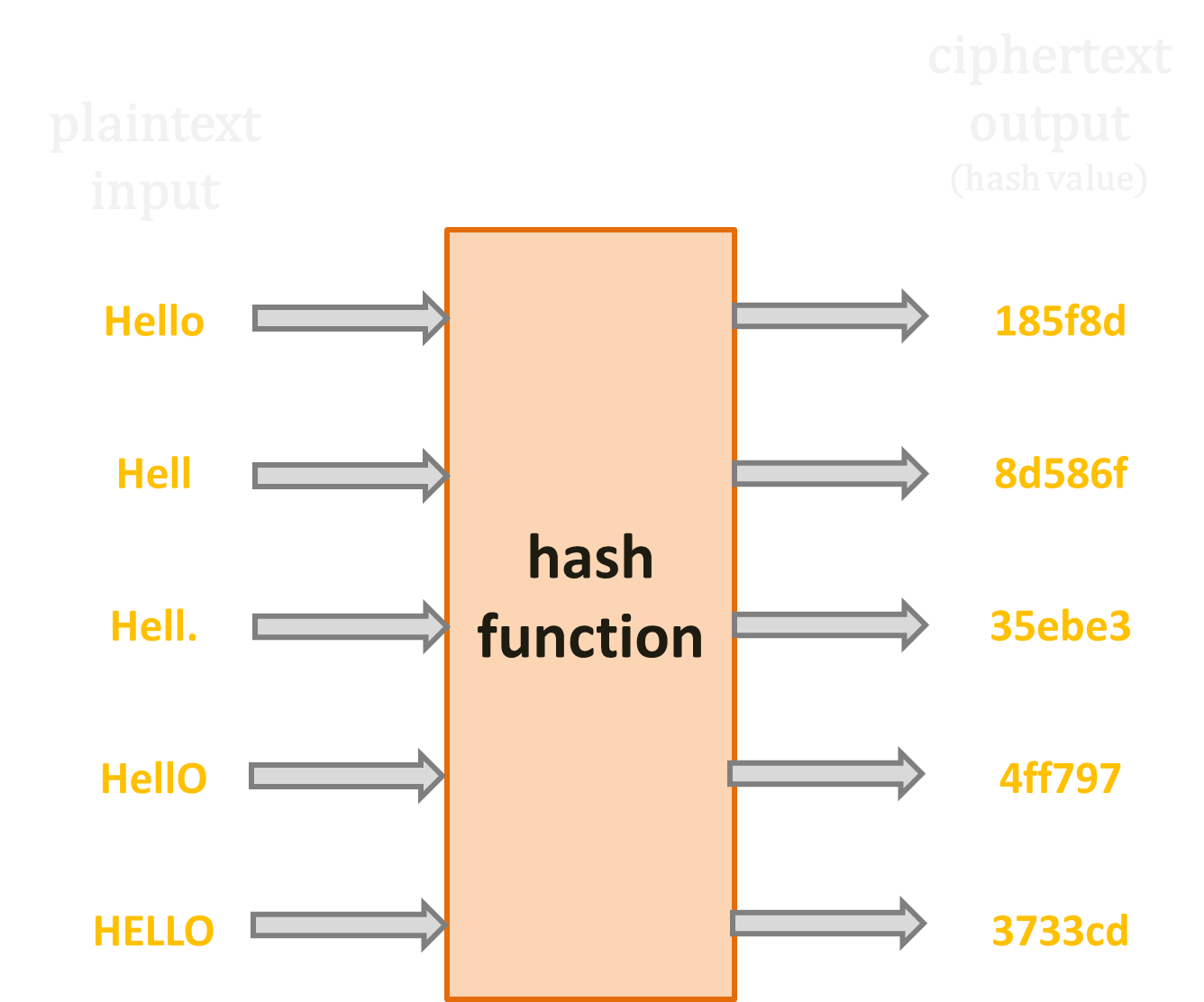### 雜湊函式運算方法

「載荷因子（load factor）」代表資料個數（設為 n）與雜湊表大小（設為 m）的比例，其值越大，越容易產生多筆資料放在同一個空間的「雜湊衝突（hash collision）」現象。如果載荷因子大於 1，根據「鴿巢原理（pigeonhole principal）」，雜湊衝突將不可避免。#### 除法（division method）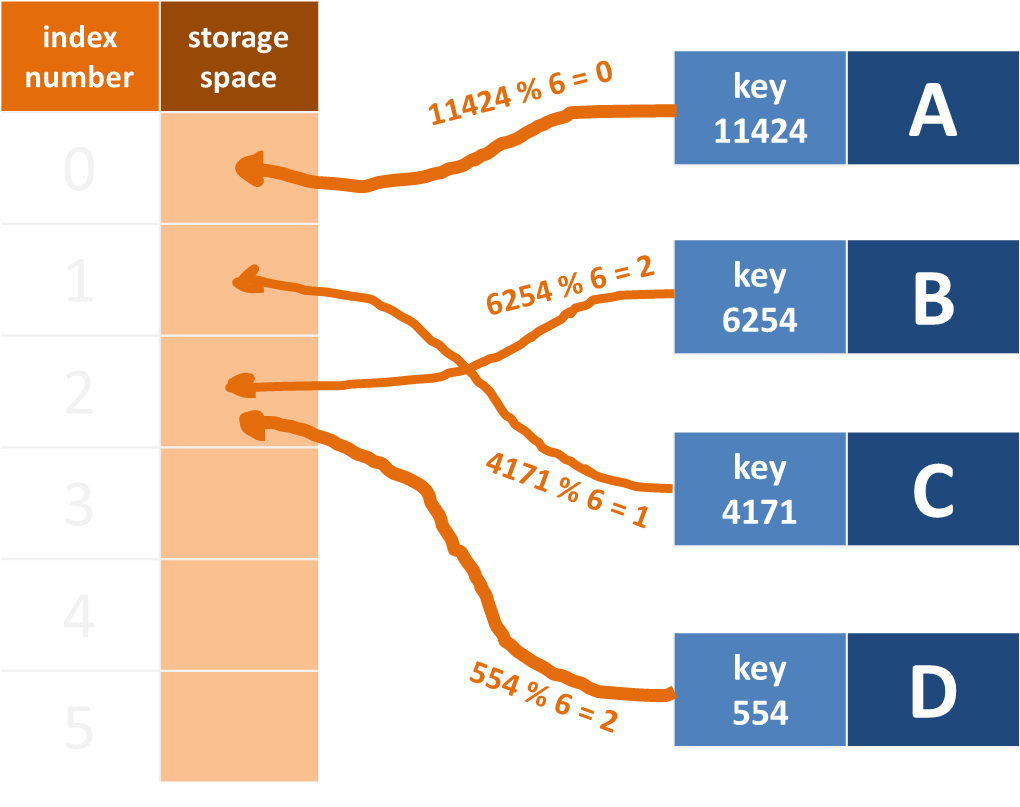#### 乘法（multiplication method）1. 將「key」值乘上一個小於 1 的無理數 A（常用 (√5–1)/2），得一個更大的無理數。
2. 將步驟 1. 求得的大無理數模除 1，去掉整數部分，剩下小數。
3. 將 m 乘以步驟 2. 求出的小數，將小數點右移，得界於 0~(m–1) 之間的無理數。
4. 將步驟 3. 求出的無理數取高斯符號，得界於 0~(m–1) 之間的整數，這個整數就是「key」值的索引值。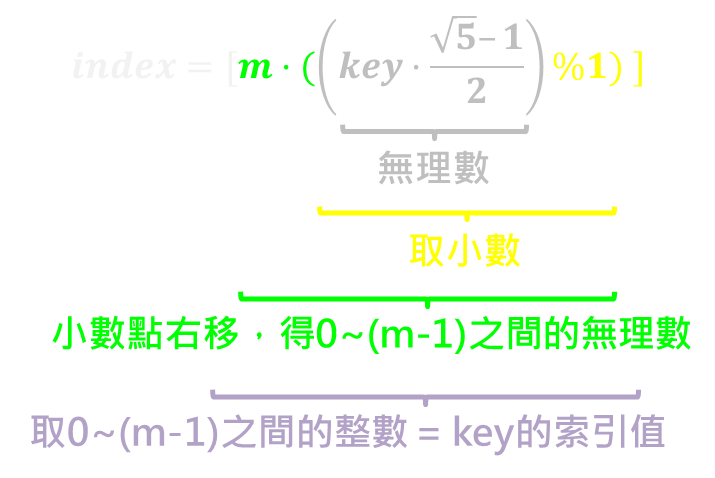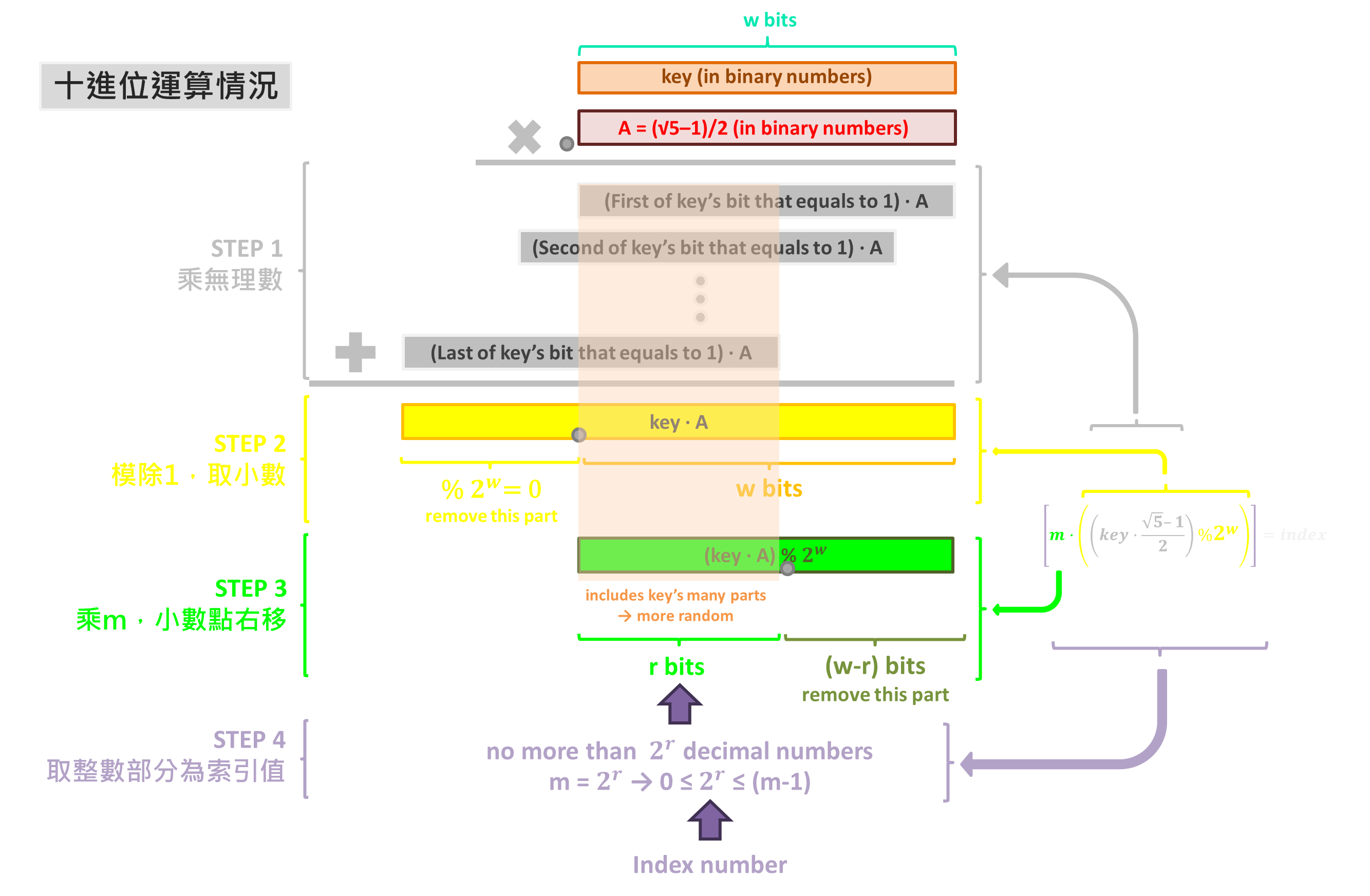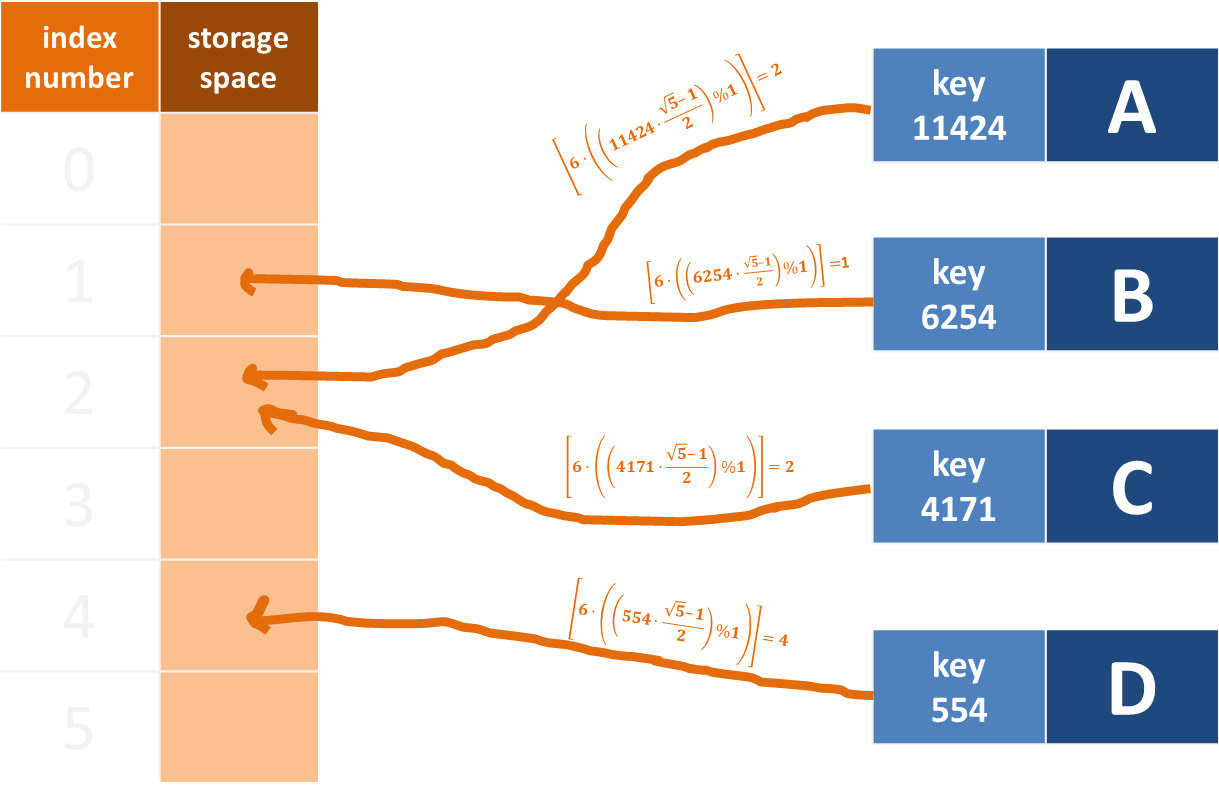## 處理雜湊衝突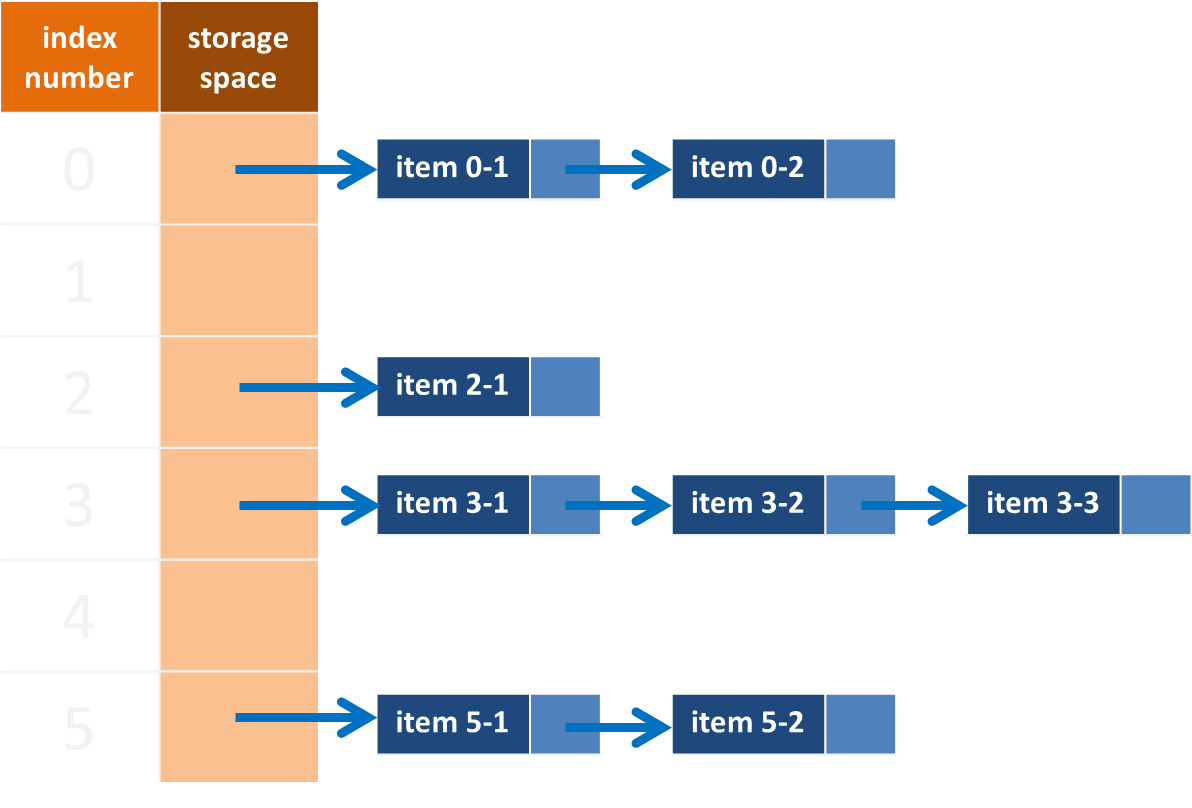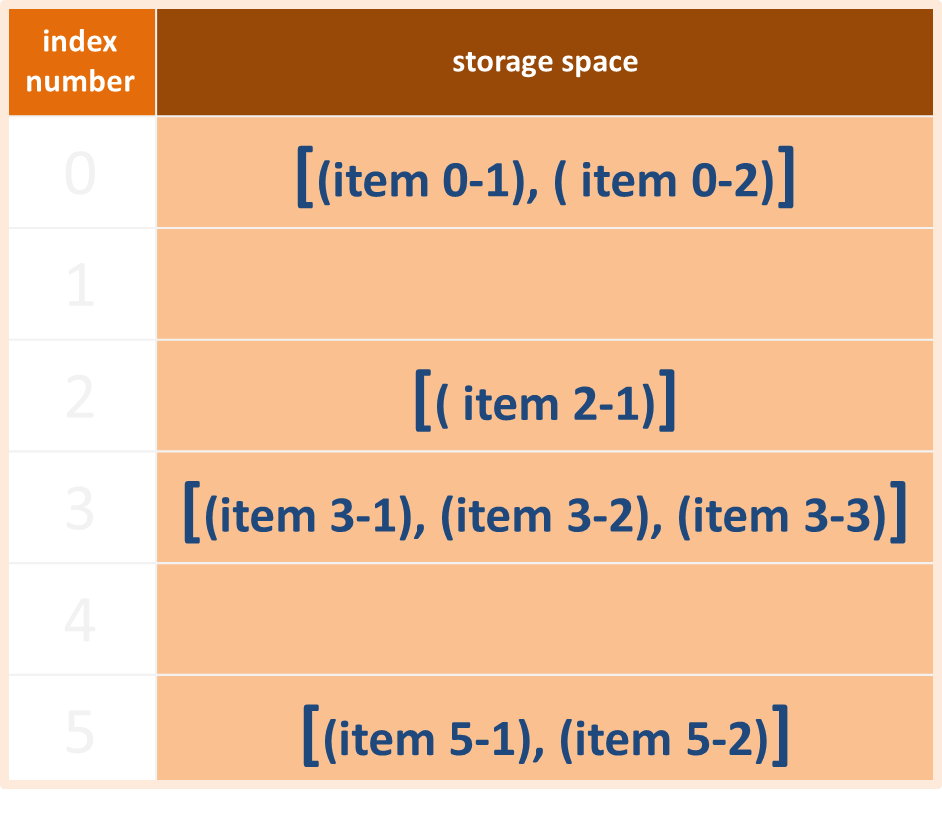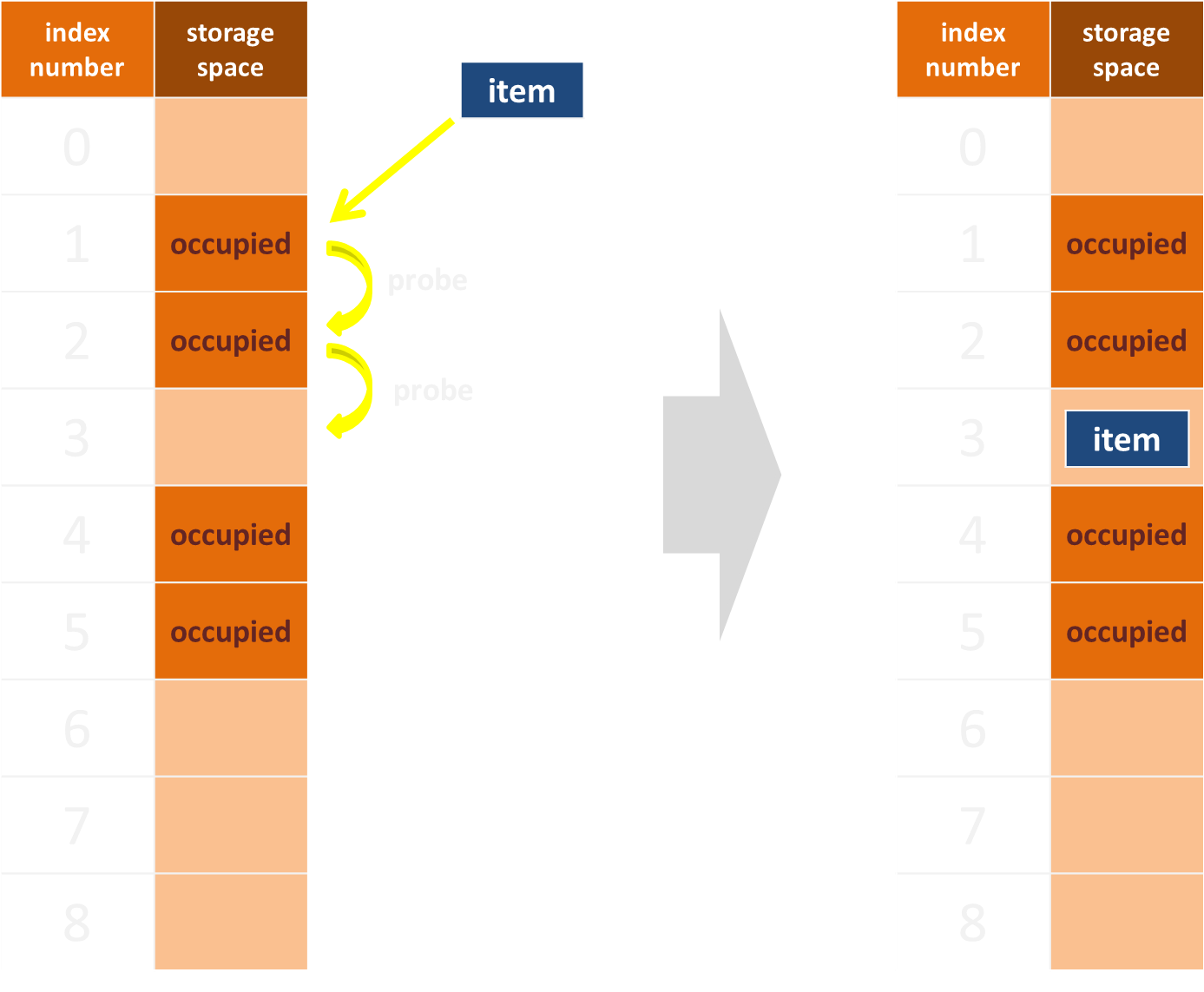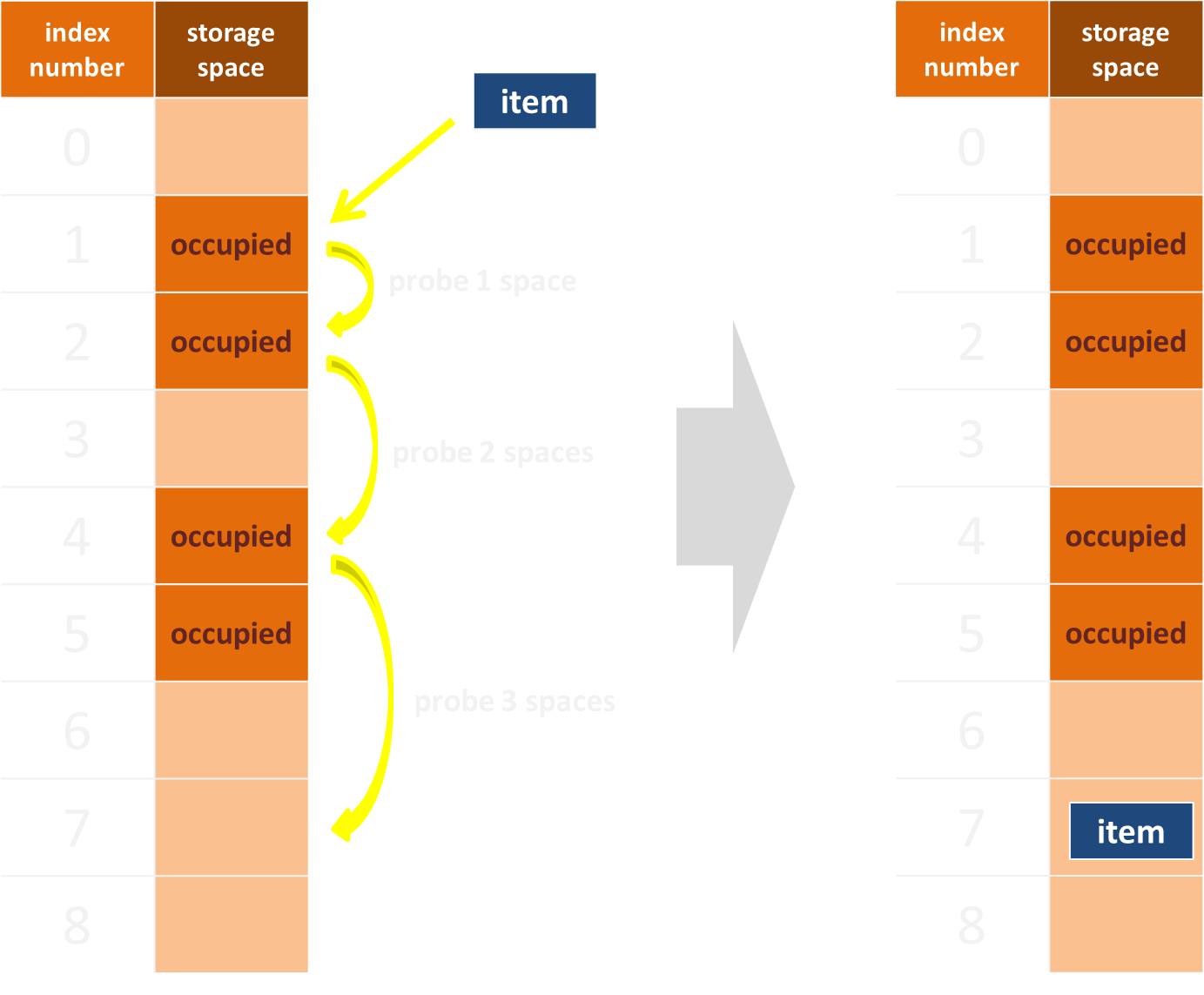1. 第一次雜湊函式運算，得 index₁=F₁(k)。
2. 若有衝突，進行第二次雜湊運算，得 index₂=F₁(k)+1*F₂(k)。
3. 若仍有衝突，進行第三次雜湊運算，得 index₃=F₁(k)+2*F₂(k)。
4. 若仍有衝突，進行第三次雜湊運算，得 index₄=F₁(k)+3*F₂(k)。

## 程式碼範例（JavaScript）

``````class HashTable {
constructor(size){
this.size = size;
this.table = [];

//要在table這個陣列中每個索引值對應的位置都再放入小陣列
for(let i=0; i<this.size; i++){
this.table.push([]);
}
}

//以除法實現雜湊函式
hash1(key){
return key%(this.size);
}

//以乘法實現雜湊函式
hash2(key){
let A = (Math.sqrt(5)-1)/2;
return Math.floor((this.size)*((key*A)%1));
}

//將一筆key-value pair放進雜湊表
set(key, value){
let index = this.hash2(key); //用雜湊函式處理key值，設定該筆資料索引值
this.table[index].push({key,value}); //以物件形式將key-value pair放進對應索引位置
}

//輸入key值，從雜湊表取得對應的value
get(key){
let index = this.hash2(key); //用雜湊函式處理key值，找到該筆資料索引值

//因可能有雜湊衝突，即同一個索引位置有不只一筆資料，因此用for迴圈在該索引位置內跑，找到與輸入key值相符者才是正確資料
for(let i = 0; i < this.table[index].length; i++){
if(this.table[index][i].key === key){
console.log(this.table[index][i]);
return;
}
}
console.log("not in this table") //如果雜湊表中找不到對應資料，則顯示"not in this table"
}

//顯示雜湊表
printAll(){
console.log(this.table);
}
}
``````

## 時間複雜度

1. 平均&最佳情況：O(1)，在多數情況下，可透過雜湊函式求「key」值，直接找到對應位置並進行操作。
2. 最差情況：O(n)，若產生雜湊衝突且使用「封閉循址法」處理，則在同一個「key」值的儲存空間中要再做一次搜尋。

## 空間複雜度

1. 封閉循址法：O(m+n) = O(n)，原本雜湊表的大小為 m，但另外需要 n 個儲存空間處理對應到同一索引值的資料。
2. 開放定址法：O(n)，資料透過不斷探測，在原雜湊表中找到空的位置儲存，所有資料佔據的總空間大小不變。

#資料結構 #雜湊表